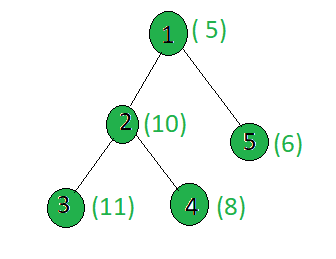Related Articles
Count the nodes of the given tree whose weight has X as a factor
• Last Updated : 13 May, 2020

Given a tree, and the weights of all the nodes, the task is to count the nodes whose weights are divisible by x.

Examples:

Input:x = 5
Output: 2
Only the nodes 1 and 2 have weights divisible by 5.

## Recommended: Please try your approach on {IDE} first, before moving on to the solution.

Approach: Perform dfs on the tree and for every node, check if it’s weight is divisible by x or not. If yes then increment the count.

Implementation:

## C++

 `// C++ implementation of the approach``#include ``using` `namespace` `std;`` ` `long` `ans = 0;``int` `x;``vector<``int``> graph;``vector<``int``> weight(100);`` ` `// Function to perform dfs``void` `dfs(``int` `node, ``int` `parent)``{`` ` `    ``// If weight of the current node``    ``// is divisible by x``    ``if` `(weight[node] % x == 0)``        ``ans += 1;`` ` `    ``for` `(``int` `to : graph[node]) {``        ``if` `(to == parent)``            ``continue``;``        ``dfs(to, node);``    ``}``}`` ` `// Driver code``int` `main()``{``    ``x = 5;`` ` `    ``// Weights of the node``    ``weight = 5;``    ``weight = 10;``    ``weight = 11;``    ``weight = 8;``    ``weight = 6;`` ` `    ``// Edges of the tree``    ``graph.push_back(2);``    ``graph.push_back(3);``    ``graph.push_back(4);``    ``graph.push_back(5);`` ` `    ``dfs(1, 1);`` ` `    ``cout << ans;`` ` `    ``return` `0;``}`

## Java

 `// Java implementation of the approach ``import` `java.util.*;`` ` `class` `GFG``{``     ` `static` `long` `ans = ``0``; ``static` `int` `x; ``static` `Vector> graph=``new` `Vector>(); ``static` `Vector weight=``new` `Vector(); `` ` `// Function to perform dfs ``static` `void` `dfs(``int` `node, ``int` `parent) ``{ `` ` `    ``// If weight of the current node ``    ``// is divisible by x ``    ``if` `(weight.get(node) % x == ``0``) ``        ``ans += ``1``; `` ` `    ``for` `(``int` `i = ``0``; i < graph.get(node).size(); i++) ``    ``{ ``        ``if` `(graph.get(node).get(i) == parent) ``            ``continue``; ``        ``dfs(graph.get(node).get(i), node); ``    ``} ``} `` ` `// Driver code ``public` `static` `void` `main(String args[])``{ ``    ``x = ``5``; `` ` `    ``// Weights of the node ``    ``weight.add(``0``); ``    ``weight.add(``5``); ``    ``weight.add(``10``);; ``    ``weight.add(``11``);; ``    ``weight.add(``8``); ``    ``weight.add(``6``); ``     ` `    ``for``(``int` `i = ``0``; i < ``100``; i++)``    ``graph.add(``new` `Vector());`` ` `    ``// Edges of the tree ``    ``graph.get(``1``).add(``2``); ``    ``graph.get(``2``).add(``3``); ``    ``graph.get(``2``).add(``4``); ``    ``graph.get(``1``).add(``5``); `` ` `    ``dfs(``1``, ``1``); `` ` `    ``System.out.println(ans); ``}``} `` ` `// This code is contributed by Arnab Kundu`

## Python3

 `# Python3 implementation of the approach ``ans ``=` `0`` ` `graph ``=` `[[] ``for` `i ``in` `range``(``100``)]``weight ``=` `[``0``] ``*` `100`` ` `# Function to perform dfs ``def` `dfs(node, parent):``    ``global` `ans,x``     ` `    ``# If weight of the current node ``    ``# is divisible by x ``    ``if` `(weight[node] ``%` `x ``=``=` `0``):``        ``ans ``+``=` `1``    ``for` `to ``in` `graph[node]:``        ``if` `(to ``=``=` `parent):``            ``continue``        ``dfs(to, node)`` ` `# Driver code ``x ``=` `5`` ` `# Weights of the node ``weight[``1``] ``=` `5``weight[``2``] ``=` `10``weight[``3``] ``=` `11``weight[``4``] ``=` `8``weight[``5``] ``=` `6`` ` `# Edges of the tree ``graph[``1``].append(``2``)``graph[``2``].append(``3``)``graph[``2``].append(``4``)``graph[``1``].append(``5``)`` ` `dfs(``1``, ``1``)``print``(ans)`` ` `# This code is contributed by SHUBHAMSINGH10`

## C#

 `// C# implementation of the approach``using` `System;``using` `System.Collections.Generic;`` ` `class` `GFG``{``     ` `static` `long` `ans = 0; ``static` `int` `x; ``static` `List> graph = ``new` `List>(); ``static` `List<``int``> weight = ``new` `List<``int``>(); `` ` `// Function to perform dfs ``static` `void` `dfs(``int` `node, ``int` `parent) ``{ `` ` `    ``// If weight of the current node ``    ``// is divisible by x ``    ``if` `(weight[node] % x == 0) ``        ``ans += 1; `` ` `    ``for` `(``int` `i = 0; i < graph[node].Count; i++) ``    ``{ ``        ``if` `(graph[node][i] == parent) ``            ``continue``; ``        ``dfs(graph[node][i], node); ``    ``} ``} `` ` `// Driver code ``public` `static` `void` `Main(String []args)``{ ``    ``x = 5; `` ` `    ``// Weights of the node ``    ``weight.Add(0); ``    ``weight.Add(5); ``    ``weight.Add(10);; ``    ``weight.Add(11);; ``    ``weight.Add(8); ``    ``weight.Add(6); ``     ` `    ``for``(``int` `i = 0; i < 100; i++)``    ``graph.Add(``new` `List<``int``>());`` ` `    ``// Edges of the tree ``    ``graph.Add(2); ``    ``graph.Add(3); ``    ``graph.Add(4); ``    ``graph.Add(5); `` ` `    ``dfs(1, 1); `` ` `    ``Console.WriteLine(ans); ``}``}`` ` `// This code contributed by Rajput-Ji`
Output:
```2
```

Complexity Analysis:

• Time Complexity: O(N).
In DFS, every node of the tree is processed once and hence the complexity due to the DFS is O(N) when there are total N nodes in the tree. Therefore, the time complexity is O(N).
• Auxiliary Space: O(1).
Any extra space is not required, so the space complexity is constant.

Attention reader! Don’t stop learning now. Get hold of all the important DSA concepts with the DSA Self Paced Course at a student-friendly price and become industry ready.  To complete your preparation from learning a language to DS Algo and many more,  please refer Complete Interview Preparation Course.

In case you wish to attend live classes with industry experts, please refer Geeks Classes Live and Geeks Classes Live USA

My Personal Notes arrow_drop_up